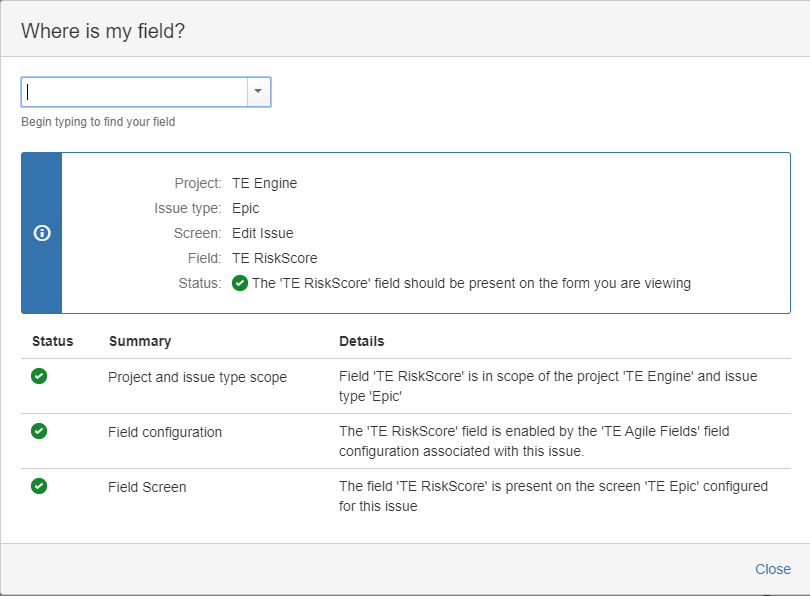cancel
Showing results for
Did you mean:
See all
See all
##### Groups
Explore all groups

# It's not the same without you

Join the community to find out what other Atlassian users are discussing, debating and creating.# MyGroovy custom calculated field created and compiles, but does not show up on the Issue type.

I've got MyGroovy add-on, similar to Scriptrunner, and I've been trying to use it to create a scripted custom field "risk" which calculates the value based on

Impact X Probability - both these fields are single list type custom fields.

The following is the groovy script I've used, which compiles fine, but the field is not appearing on the IssueType:

It is currently associated to screens for Epic, Story and Bugs. Am I missing some step in between? Any help is greatly appreciated!

===================||======================

import com.atlassian.jira.issue.Issue
import com.atlassian.jira.issue.MutableIssue
import com.atlassian.jira.issue.IssueManager
import com.atlassian.jira.component.ComponentAccessor
import com.atlassian.jira.ComponentManager
import com.atlassian.jira.issue.fields.CustomField
import com.atlassian.jira.issue.CustomFieldManager
import java.lang.Object
import java.lang.Double
import org.apache.log4j.Logger
import org.apache.log4j.Level

def log = Logger.getLogger("com.onresolve.jira.groovy")
log.setLevel(Level.DEBUG)

def impact = ComponentAccessor.getCustomFieldManager().getCustomFieldObjectsByName("TE Business Impact").toString()

def probability = ComponentAccessor.getCustomFieldManager().getCustomFieldObjectsByName("TE Technical Complexity").toString()

def risk

if ((impact!="null") && (probability!="null")) {
risk = Double.parseDouble(impact) * Double.parseDouble(probability)
} else {
risk = null
}

return risk

#### 1 accepted

This is how we managed to get it working at Collinson:

import com.atlassian.jira.component.ComponentAccessor
import java.lang.Double

// needed to get custom fields
def customFieldManager = ComponentAccessor.getCustomFieldManager()

// get generic custom fields
def impact = customFieldManager.getCustomFieldObjectsByName("TE Business Impact").first()
def probability = customFieldManager.getCustomFieldObjectsByName("TE Technical Complexity").first()

// get instances for this issue
def impactValue = issue.getCustomFieldValue(impact)
def probabilityValue = issue.getCustomFieldValue(probability)

// get actual selected values
def selectedImpactValue = ((LazyLoadedOption)impactValue).getValue() as Double
def selectedProbabilityValue = ((LazyLoadedOption)probabilityValue).getValue() as Double

// calculate risk
def riskValue = null

if (selectedImpactValue && selectedProbabilityValue) {
riskValue = selectedImpactValue * selectedProbabilityValue
}

return riskValue

Hello,

You're not getting values this way:

`ComponentAccessor.getCustomFieldManager().getCustomFieldObjectsByName("TE Business Impact").toString()`

You're only getting the custom field. So your script is likely throwing errors.

Your script should look something like this:

`import com.atlassian.jira.issue.customfields.option.Optionimport com.atlassian.jira.issue.CustomFieldManager@StandardModuleCustomFieldManager customFieldManagerOption impact = (Option) issue.getCustomFieldValue(customFieldManager.getCustomFieldObjectsByName("TE Business Impact").first())Option probability = (Option) issue.getCustomFieldValue(customFieldManager.getCustomFieldObjectsByName("TE Technical Complexity").first())if ((impact != null) && (probability != null)) {    //assuming you want to parse names of options as numbers    return Double.parseDouble(impact.value) * Double.parseDouble(probability.value)}return null `

This is how we managed to get it working at Collinson:

import com.atlassian.jira.component.ComponentAccessor
import java.lang.Double

// needed to get custom fields
def customFieldManager = ComponentAccessor.getCustomFieldManager()

// get generic custom fields
def impact = customFieldManager.getCustomFieldObjectsByName("TE Business Impact").first()
def probability = customFieldManager.getCustomFieldObjectsByName("TE Technical Complexity").first()

// get instances for this issue
def impactValue = issue.getCustomFieldValue(impact)
def probabilityValue = issue.getCustomFieldValue(probability)

// get actual selected values
def selectedImpactValue = ((LazyLoadedOption)impactValue).getValue() as Double
def selectedProbabilityValue = ((LazyLoadedOption)probabilityValue).getValue() as Double

// calculate risk
def riskValue = null

if (selectedImpactValue && selectedProbabilityValue) {
riskValue = selectedImpactValue * selectedProbabilityValue
}

return riskValue

This is how we managed to get it working at Collinson:

import com.atlassian.jira.component.ComponentAccessor
import java.lang.Double

// needed to get custom fields
def customFieldManager = ComponentAccessor.getCustomFieldManager()

// get generic custom fields
def impact = customFieldManager.getCustomFieldObjectsByName("TE Business Impact").first()
def probability = customFieldManager.getCustomFieldObjectsByName("TE Technical Complexity").first()

// get instances for this issue
def impactValue = issue.getCustomFieldValue(impact)
def probabilityValue = issue.getCustomFieldValue(probability)

// get actual selected values
def selectedImpactValue = ((LazyLoadedOption)impactValue).getValue() as Double
def selectedProbabilityValue = ((LazyLoadedOption)probabilityValue).getValue() as Double

// calculate risk
def riskValue = null

if (selectedImpactValue && selectedProbabilityValue) {
riskValue = selectedImpactValue * selectedProbabilityValue
}

return riskValue

Also just to add - I've checked the settings as below, the scripted field name is 'TE RiskScore' and contains the above script:This is how we managed to get it working at Collinson:

import com.atlassian.jira.component.ComponentAccessor
import java.lang.Double

// needed to get custom fields
def customFieldManager = ComponentAccessor.getCustomFieldManager()

// get generic custom fields
def impact = customFieldManager.getCustomFieldObjectsByName("TE Business Impact").first()
def probability = customFieldManager.getCustomFieldObjectsByName("TE Technical Complexity").first()

// get instances for this issue
def impactValue = issue.getCustomFieldValue(impact)
def probabilityValue = issue.getCustomFieldValue(probability)

// get actual selected values
def selectedImpactValue = ((LazyLoadedOption)impactValue).getValue() as Double
def selectedProbabilityValue = ((LazyLoadedOption)probabilityValue).getValue() as Double

// calculate risk
def riskValue = null

if (selectedImpactValue && selectedProbabilityValue) {
riskValue = selectedImpactValue * selectedProbabilityValue
}

return riskValue

Community showcase

### The add-in you’ve been waiting for: Jira Cloud for Excel 🙌

Introducing Jira Cloud for Excel Here at the product integrations team at Atlassian, we are thrilled to announce the new Jira Cloud for Excel add-in! This add-in lets you export Jira data directly ...

839 views 5 22

### Community Events

Connect with like-minded Atlassian users at free events near you!

Connect with like-minded Atlassian users at free events near you!

Unfortunately there are no Community Events near you at the moment.